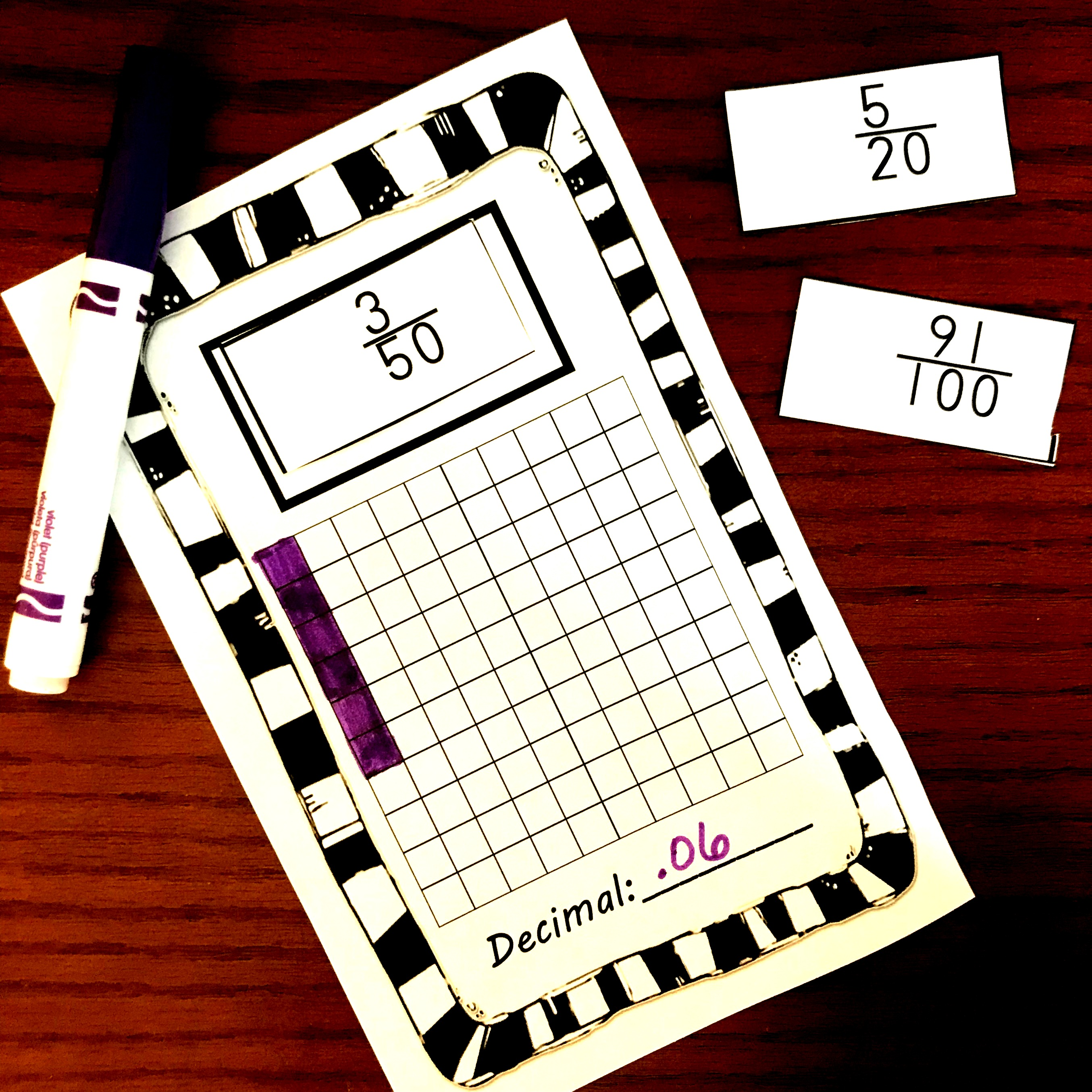Home » Math » FREE Printable and Low-Prep Fraction To Decimal Game

FREE Printable and Low-Prep Fraction To Decimal Game

This free fraction to decimal game is a perfect way for children to practice converting fractions like 1/2, 3/4, 4/5, and 5/7 to decimals.Games in a math class are incredibly important! They get children practicing a skill over and over without the boredom of a worksheet! And this game gets children working on converting fractions to decimals in a simple but fun game.

Prep – Work:

Just a little prep work is needed to get this game up and running.

1. Print off the game boards and the fraction cards
2. Next, cut out the fraction cards.
3. Finally, gather up game markers that are in two different colors, (I love using snap cubes) and you are ready to go!

How to Play Fraction to Decimal GameThis game is played just like the traditional BINGO game we all know and love!

1. Begin by passing out a fraction card out to each player.
2. Then, have them convert the fraction into a decimal. If the decimal is not exact they need to round to the thousandths place.
3. Next, have them mark the answer on their bingo cards.
4. Simply, pick up the cards, and pass another card to each player.
5. The game is over when someone has five in a row.

How to Change Fractions to Decimals

So there are a couple of different ways to change fractions to decimal.

The first way is to use an antilogarithm. If you taught children how to divide two whole numbers that have a fraction as an answer, you have already laid the background knowledge for this.  A fraction is basically a division problem. The numerator becomes my dividend (the number being divided up) and the denominator is my divisor (the number of equal parts I will divide my dividend into).

If I wanted to find the decimal for 5/7. My five goes inside the division sign, while the 7 is on the outside. Now we divide by adding a decimal point and some zeros. 5 ÷ 7 = .714285 and if I round to the nearest thousandths place my answer is .714

Decimal Grids

You know me! If it is possible, I’m going to model this out for my children. And this can be done with certain fractions.

If you can create an equivalent fraction that has a denominator of 100, children can model this on a decimal grid.In this example, we are trying to find the decimal for 3/50. If I find the equivalent fraction, I can see that 3/50 = 6/100. I color in 6 out of the 100 girds and now it is easy to see what my decimal is. I don’t have any tenths, but I have six hundredths. So my answer is 0.06.

(These task cards are apart of my fraction to decimal bundle)Converting fractions to decimals is not a difficult skill, but it needs to be practiced. And this simple fraction to decimal game gets our kiddos working on this skill.

Enjoy!

You’ve Got This

Rachel